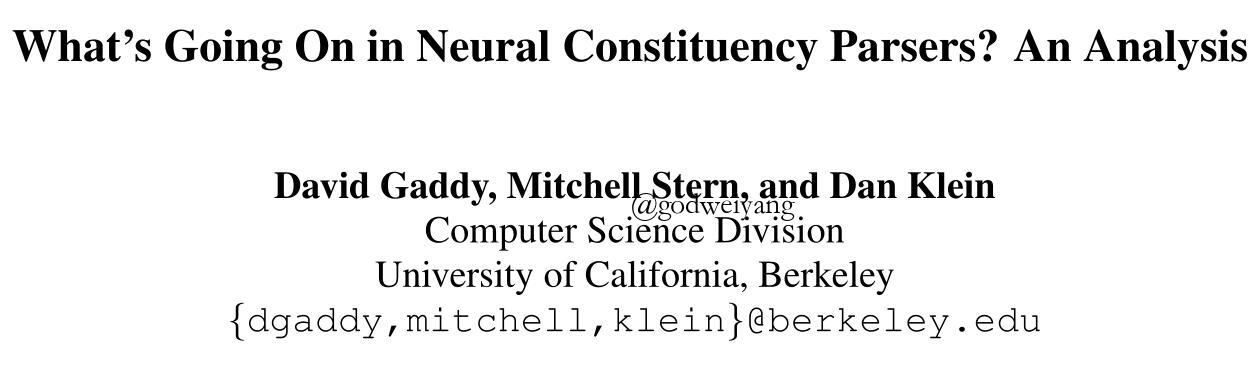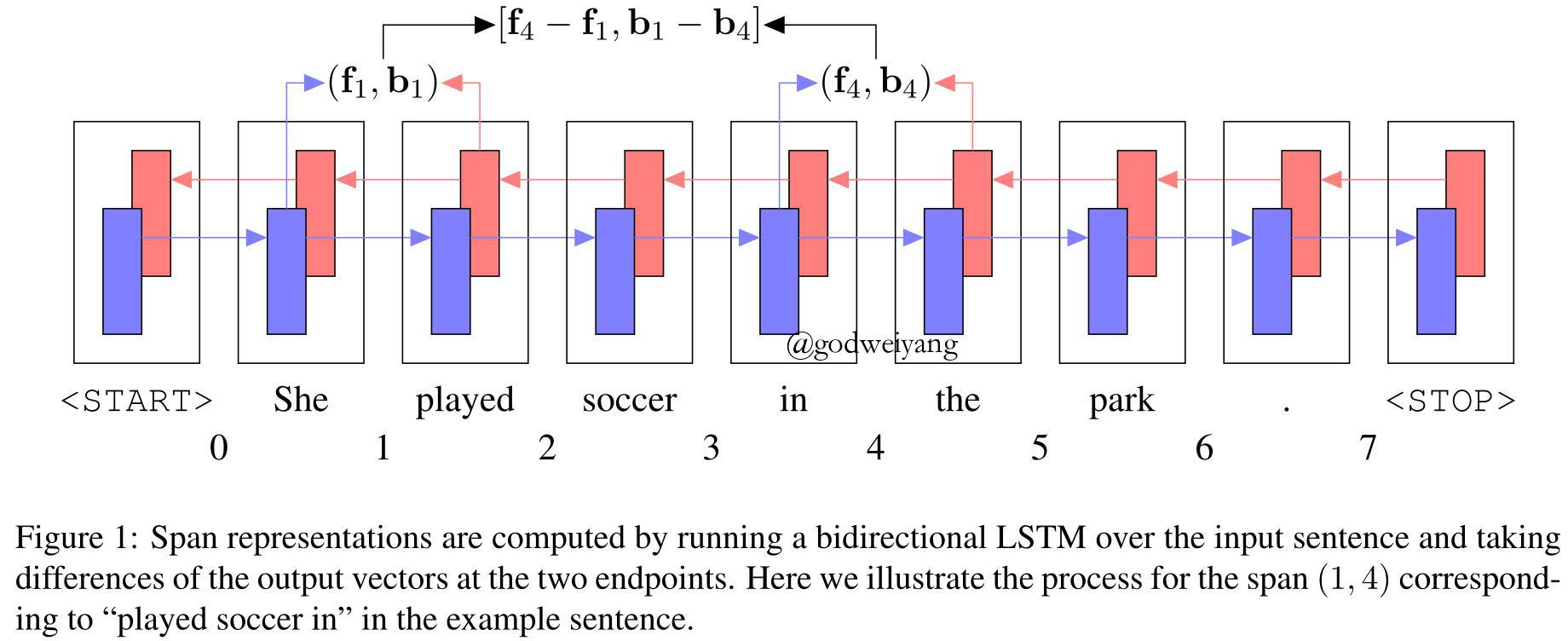What's Going On in Neural Constituency Parsers? An Analysis# 句法分析模型

## 模型总览

$s(T) = \sum\limits_{(i,j,l) \in T} {s(i,j,l)}$

$\hat T = \mathop {\arg \max }\limits_T s(T)$

## 短语表示

${ {\bf{r}}_{ij}} = [{ {\bf{f}}_j} - { {\bf{f}}_i},{ {\bf{b}}_i} - { {\bf{b}}_j}]$## 短语类别得分

$s(i,j,l) = {[{ {\bf{W}}_2}ReLU({ {\bf{W}}_1}{ {\bf{r}}_{ij}} + { {\bf{z}}_1}) + { {\bf{z}}_2}]_l}$

## 句法分析

${s_{best}}(i,i + 1) = \mathop {\max }\limits_l s(i,i + 1,l)$

${s_{best}}(i,j) = \mathop {\max }\limits_l s(i,j,l) + \mathop {\max }\limits_k [{s_{best}}(i,k) + {s_{best}}(k,j)]$

## 模型训练

$\mathcal L = \max \left( {0,\mathop {\max }\limits_T [s(T) + \Delta (T,{T^*})] - s({T^*})} \right)$

## 实验结果

转载规则

《What's Going On in Neural Constituency Parsers? An Analysis》 韦阳 采用 知识共享署名 4.0 国际许可协议 进行许可。
上一篇Constituency Parsing with a Self-Attentive Encoder

2018-07-04A Minimal Span-Based Neural Constituency Parser

2018-06-28
目录